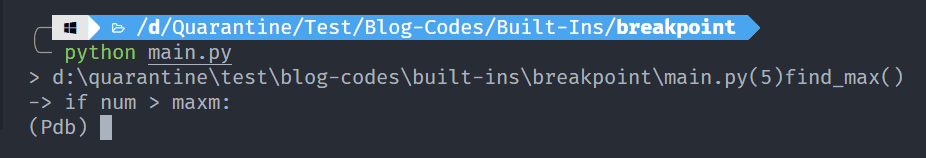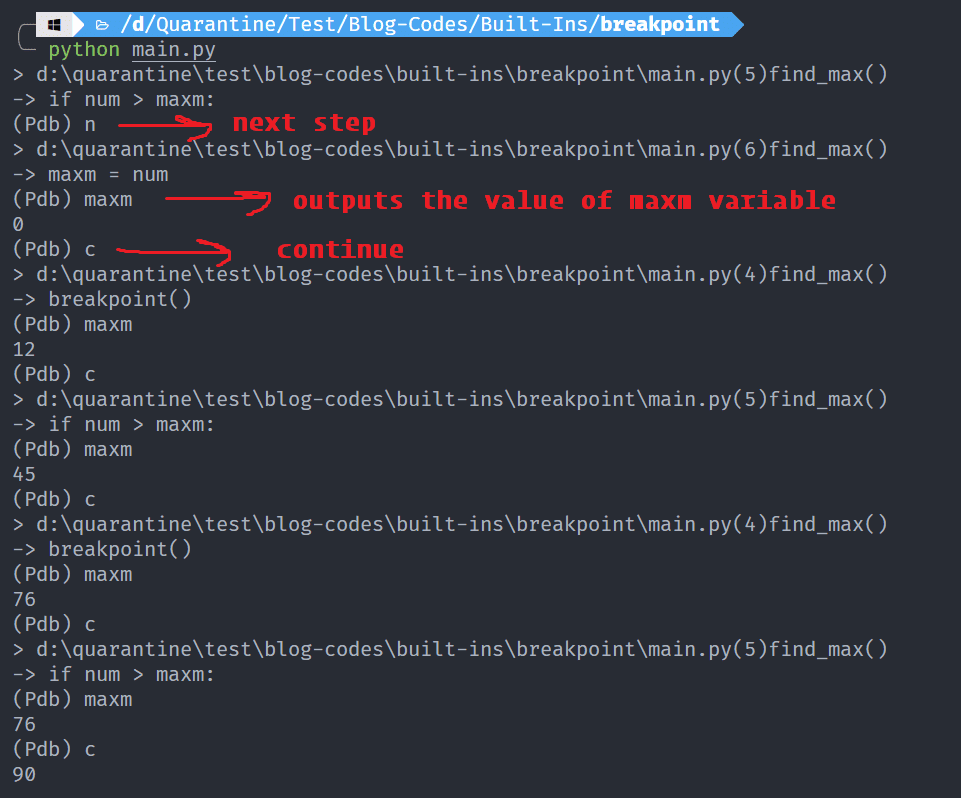# Ashutosh Writes# breakpoint() function in Python

## Introduction

While writing Python code, you will often need to debug the code. There are several tools such as debuggers and linters available but Python itself has a built-in function for this purpose called `breakpoint()`.

The `breakpoint()` function was introduced in Python 3.7 version. Before its introduction, we used to use a Python module called Python Debugger. It has to be imported and then called upon as below:

``````import pdb
pdb.set_trace()
``````

But with the introduction of `breakpoint()` function, we can call on it inside the script we want to debug without having to import any new modules.

The syntax of `breakpoint()` function is:

``````breakpoint(*args, **kws)
``````

where `*args` and `**kws` are option arguments and keyword arguments respectively.

## List of Commands

There are various commands you can use while debugging. Some of them are:

• `h`: Help
• `w`: where
• `n`: next
• `s`: step (step into the function)
• `c`: continue
• `p`: print
• `l`: list
• `q`: quit

Once you’ve finished debugging your code you can type `"c"` to continue and exit Python’s Debugger module.

## Examples

Let us take an example of a function to find the maximum in a list.

``````def find_max(nums):
maxm = 0
for num in nums:
breakpoint()
if num > maxm:
maxm = num

return maxm

nums = [12, 45, 76, 11, 90]
print(find_max(nums))
``````

When you run the function, you get this output:The `breakpoint()` function opens the Python debugger in the console. Let us see how we can debug the function.The debugger opens at the step: `if num > maxm`. So, when we used the n command, it shows the next step, i.e., `maxm = num`. At this stage, the value of `maxm` will obviously be 0. The `c` command is used to continue the loop. After we run c, the value of `maxm` is changed.

## Conclusion

In this part, we learned about the Python `breakpoint()` function with the help of examples. There are more advanced usage of this function which is beyond the scope of this article.